Configure risk score
• 17 Jan 2022

# Configure risk score

The risk scoring topic gives a high-level overview of what likelihood and impact means in Traceable and how the risk score is calculated.

The risk score of an API Endpoint helps you to prioritize your resources in securing the APIs. A risk score is a dynamic metric which is calculated from likelihood and impact of a potential API Endpoint exploitLikelihood is the probability or chance for an API to be exploited. Impact is the effect that an API Endpoint breach may have on your infrastructure and organization.

Traceable calculates a risk score for each discovered API Endpoint. A high-risk score does not necessarily mean that the Endpoint is under attack. A high-risk score provides you an insight to prioritize compensating controls and monitoring activities.

You can customize risk score in Traceable by configuring the following:

• Configure how much each factor contributes to the likelihood and impact scores from their respective tabs.
• Modify the Risk Lookup Table or configure how the quantitative risk score translates into Risk Levels from the Scoring tab. The risk levels configure the risk as low, medium, high, and critical.

### Configurable sections in risk score

In the entire risk scoring exercise, following are the categories in which you can adjust the values:

• Values in the lookup table
• Risk level using a slider
• Enable or disable factors for likelihood along with score for each subfactor
• Enable or disable factors for impact along with score for each subfactor

## Lookup table and risk level

The lookup table maps the levels of likelihood and impact to an overall risk score value (numeric). From Traceable platform's home page, navigate to Administration (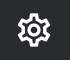) > Risk Scoring to customize the risk score.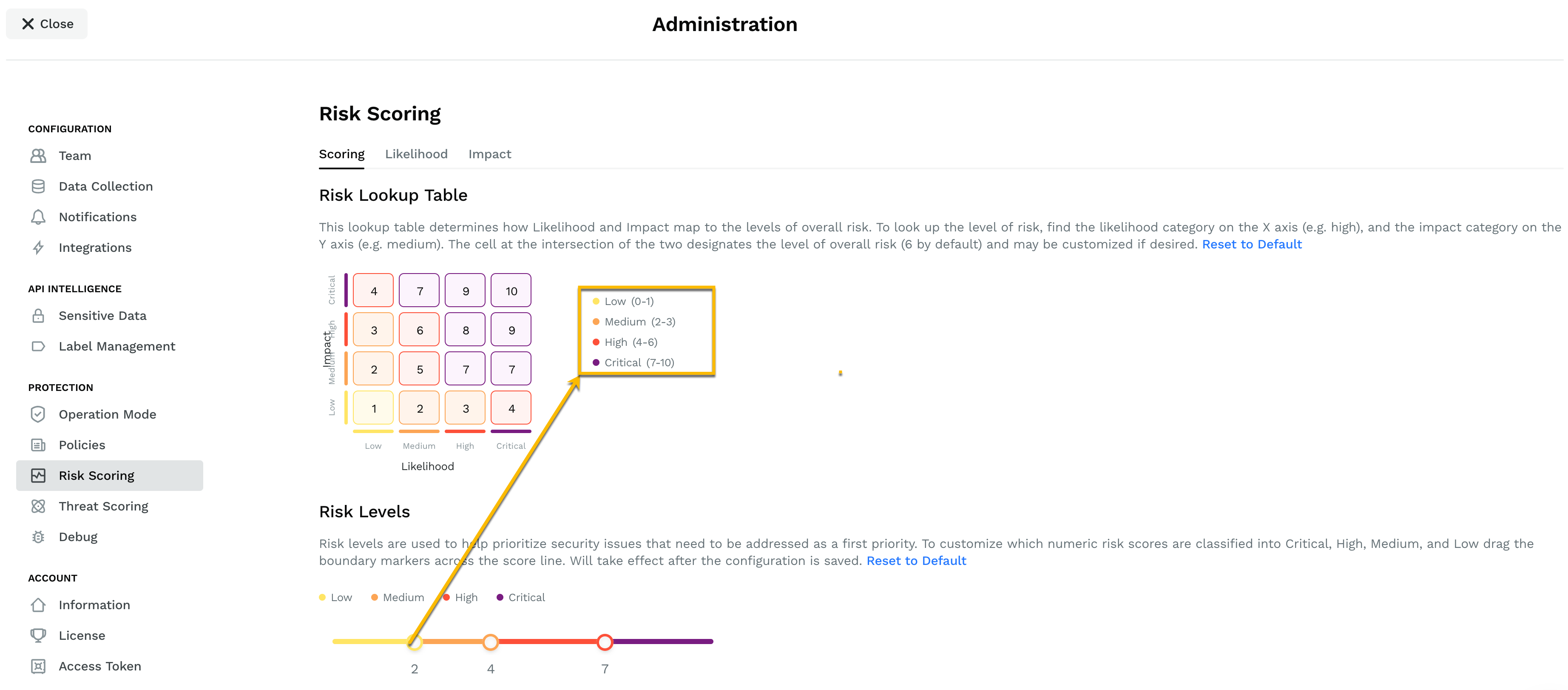Lookup table and risk level adjustment

Based on your environment and risk assessment, you can configure the risk score that you want to assign for different combinations of likelihood and impact. For example, as shown in the screenshot above, an endpoint with high likelihood and medium impact would have a risk score of 7. For more information with examples on calculating risk score, see Calculating risk score.

The risk-level slider lets you define the range of numeric risk score values for a low, medium, high, or critical risk. When you adjust the slider, the score range is update in the legend next to the lookup table as shown in the screenshot above.

## Likelihood

You can independently set the likelihood component of a risk. The likelihood, as explained earlier, is the probability of a vulnerability being exploited by a threat. Traceable provides the following factors and subfactors of likelihood.

FactorSubfactor
Exploit surface - Likelihood of an API Endpoint being exploited is directly proportional to the number of input vectors present.
• Request has no parameters
• Request has only one parameter
• Request has two to four parameters
• Request has five to 9 parameters
• Request has ten or more parameters
Motive - Probable reasons for any threat actor to exploit an API Endpoint.
• Response has sensitive data.
Ease of access - Exposure of an API Endpoint is directly proportional to the threat it faces. An external well-known API Endpoint accessible to the public is at a higher risk than an internal API Endpoint.
• Endpoint is external and restricted
• Endpoint is internal and restricted
• Endpoint is internal and popular
• Endpoint is external and popular

A popular endpoint would be an endpoint that is accessed by a wide range of users, for example, `/cart`. While a restricted endpoint would be one that has limited access, for example, `/admin`.

You can individually assign a likelihood score between 0-10 to each subfactor.

You can independently enable or disable each likelihood factor.

### Calculating likelihood score

Each subfactor has a score between 0-10. Among the subfactors, Traceable assigns the highest score among subfactors as the factor score. For example, if for the Exploit surface factor, the subfactor Request has ten or more parameters has a score of 8 and is the highest among all the subfactors, thus the score 8 is picked as the factor score. The same process is repeated for all the other factors. The following screenshot shows one of the many likelihood categories.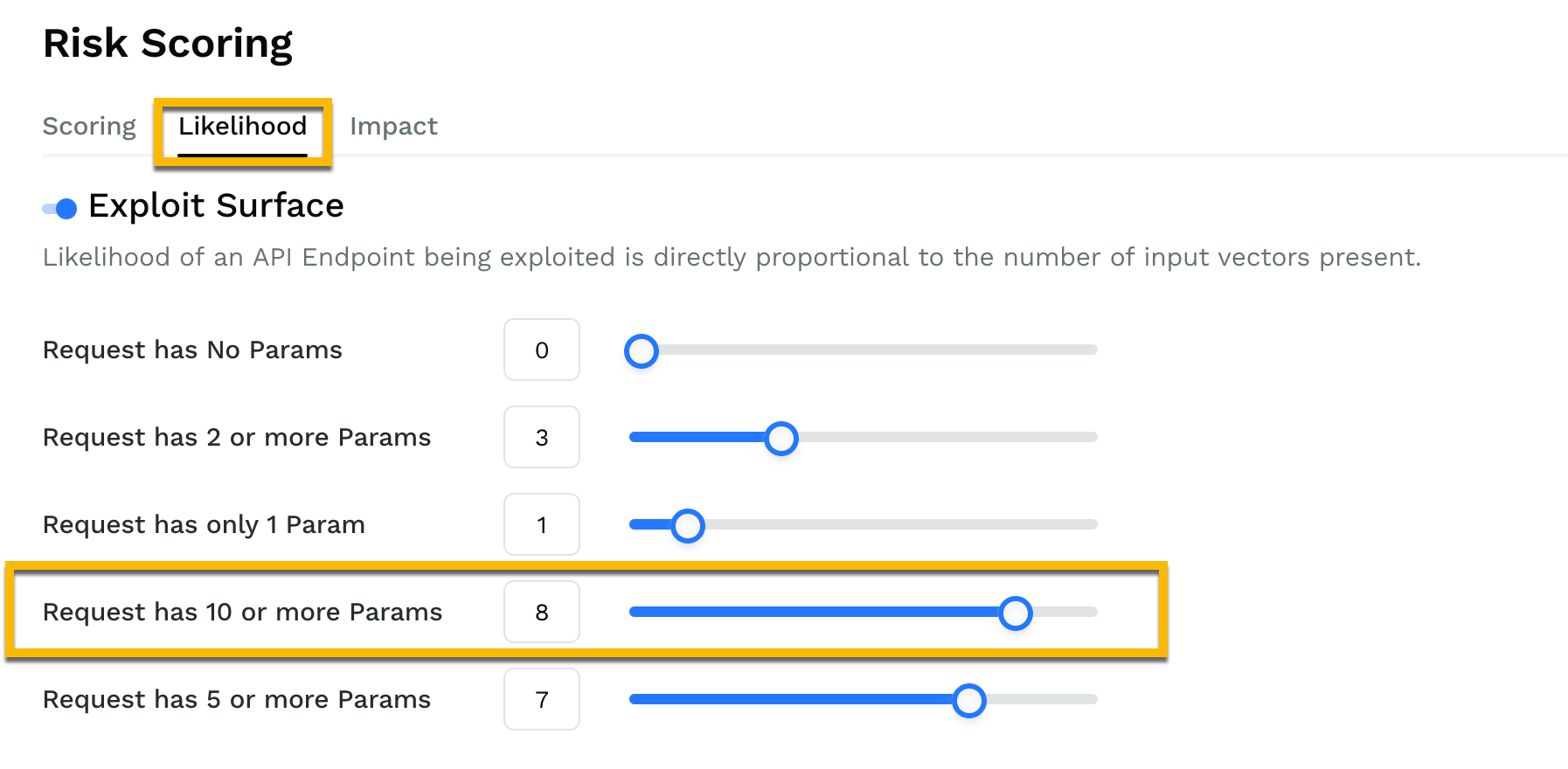The likelihood score is calculated by taking the average of the highest score of all the enabled factors.

Example

Factor

Subfactor with highest configured score

Score

Exploit surface

Request has ten or more parameters

8
Motive

Response has personally identifiable information (PII)

10

Ease of access

Endpoint is external and popular

8

Total score =8+10+8= 26

Likelihood score =26/3=8.6

A score of 8.6 indicates that there is an 86% chance that your API would be exploited for the above reasons.

Important:
The average is taken by dividing by the number of factors that are enabled. For example, in the above calculation, if only two factors were enabled (exploit surface and motive), then the likelihood score would be 9.

## Impact

Impact defines the effect a breach or exploitation of vulnerability will have your organization and business. The impact score is one of the key components in carrying out the business impact analysis (BIA) of an organization. Traceable provides the following factors and subfactors of impact.

FactorSubfactor

Sensitive data exfiltration - The type and number of sensitive data items disclosed by an API endpoint have a direct impact on the risk

• Response data contains PII >= 3
• Response data contains PII < 3

Loss of confidentiality - A breach in confidentiality of data or user authentication credentials used by an API endpoint may directly or indirectly impact the risk of exploitation.

• Unauthenticated

The impact score is calculated in the same way as likelihood score. For more information, see Calculating likelihood score.

## Tags

Tags form another likelihood and impact category. You can, based on your understanding of your APIs, assign a score between 0-10 to the tags (which act as subfactors). This score would apply to all the API Endpoint to which you apply this tag.

Traceable also gives you an option to override the likelihood score previously calculated and replace it with the score given to tags. This gives you flexibility to decide the likelihood score based on your understanding of APIs and environment.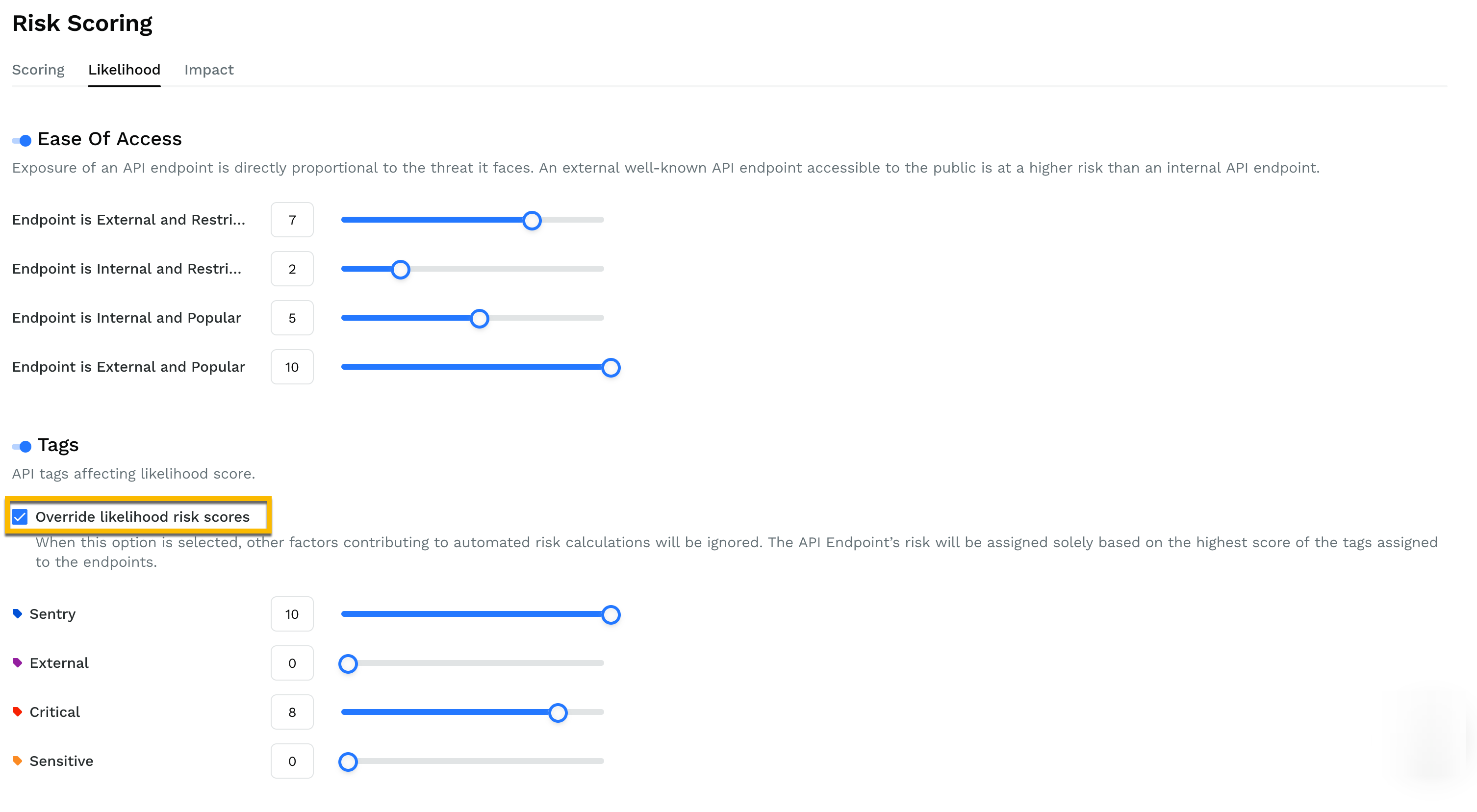## Calculating risk score

Calculating risk score for an endpoint consists of the following two steps:

1. Configure or update the lookup table. Traceable provides default values in the lookup table which will work for majority of use cases. Before you change the default values, make sure that you understand your API environment and the possible scores combinations of likelihood and impact. The values can be between 0-10.
2. Find the intersection value of likelihood and impact in the lookup table to determine the risk score.

Following are two examples to explain how Traceable calculates the risk score. Refer to the following two screenshots of likelihood and impact for both the examples.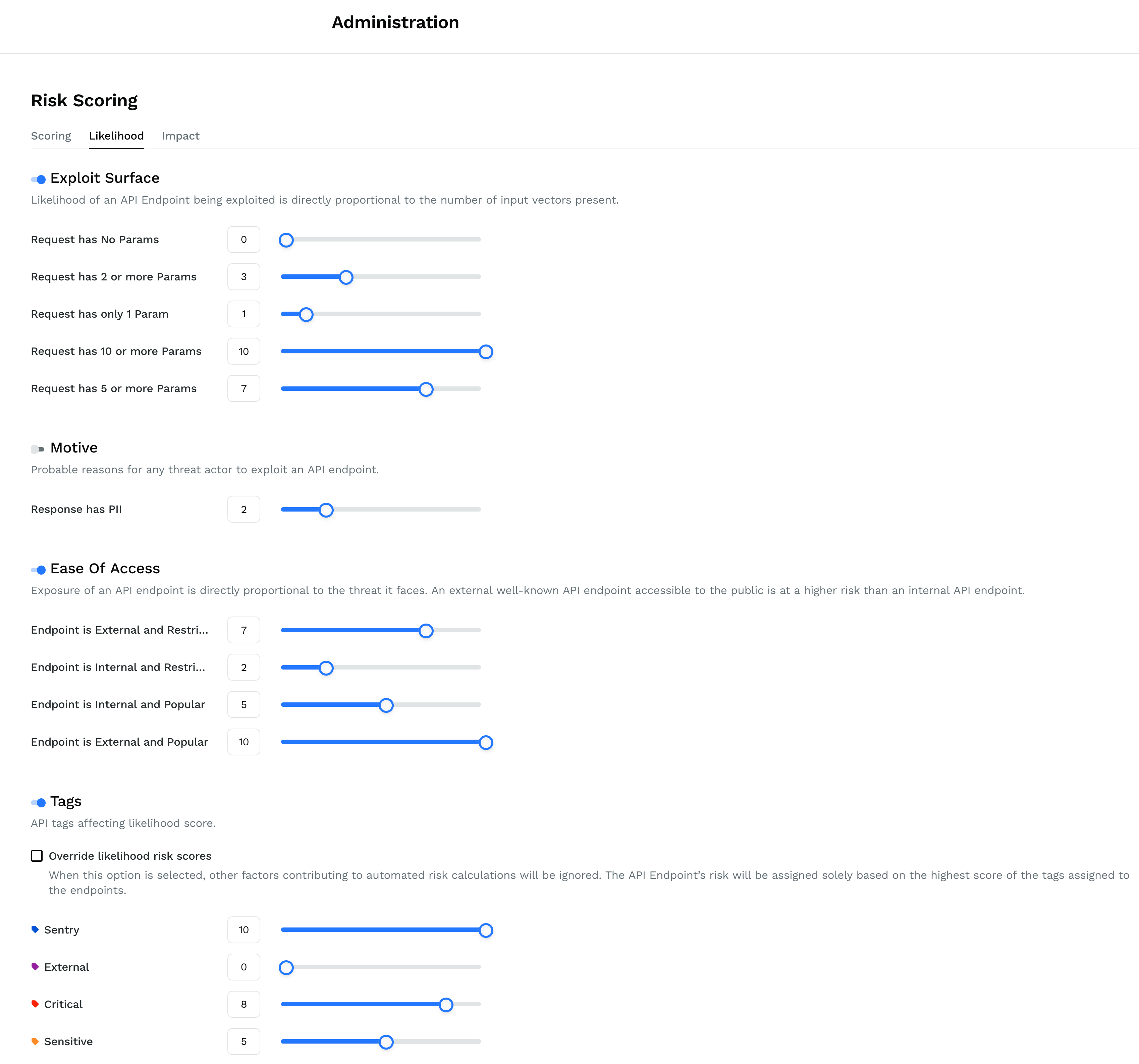Likelihood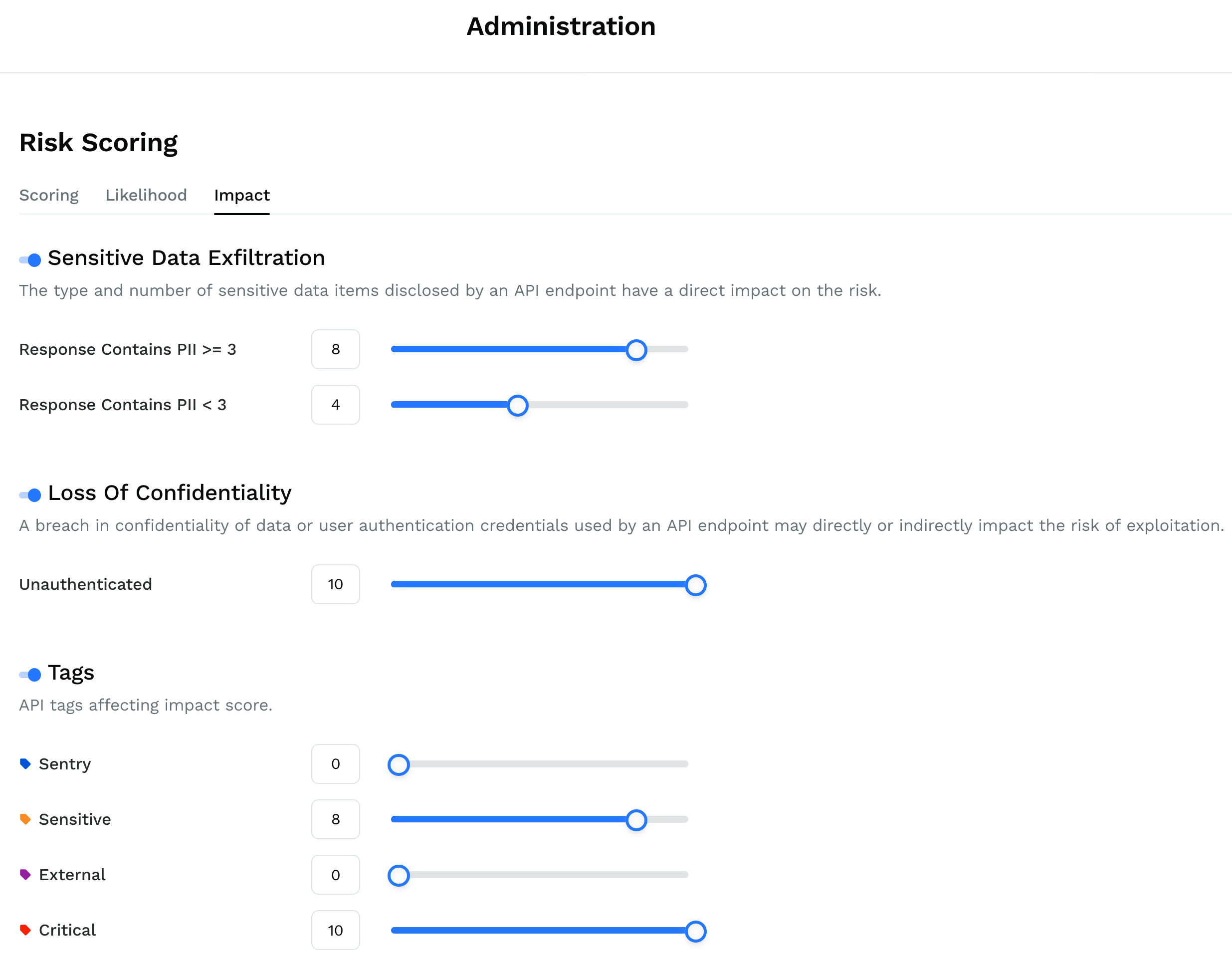Impact

Example 1 has the following assumptions for the API:

• The API is external - You can sort APIs based on External or All () filter from the API Endpoints page.
• API is popular and contains 6 request parameters.
• The API has no tags assigned to it.

You can check whether an API has any tags assigned to it from the API Endpoints home page where all the APIs are listed. The APIs which have a tag are marked (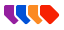) accordingly. You can also click on an API to view the assigned tag. Click on Manage Labels in the API Endpoints page.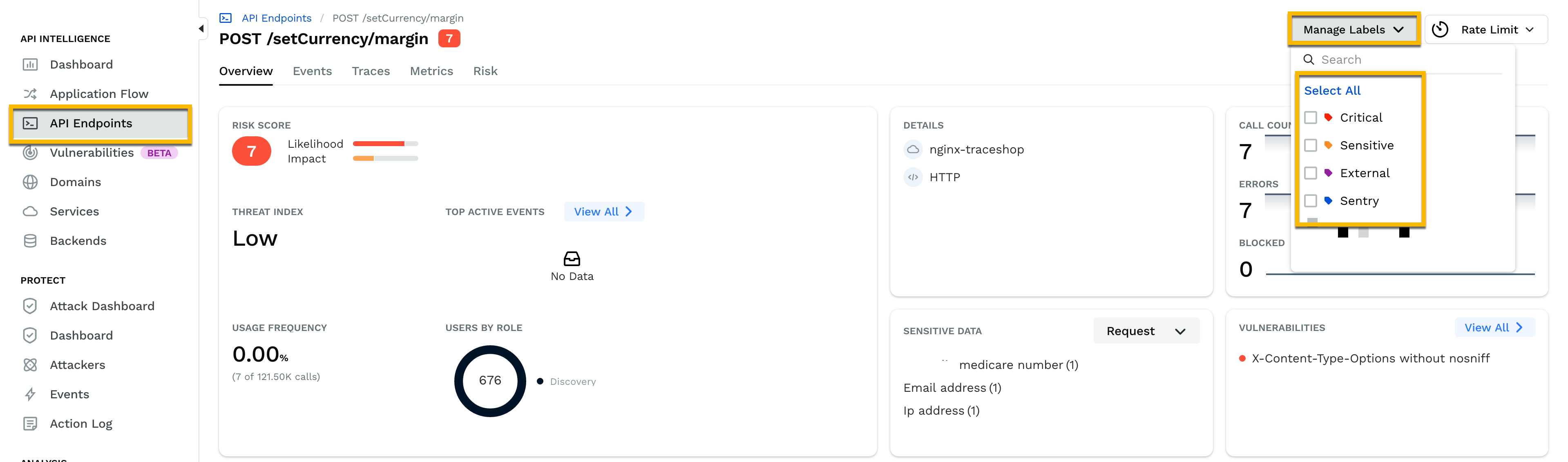The following table describes risk score calculation:

LikelihoodImpact

Total no. of factors = 3 (Motive is disabled)

Total no. of factors = 3

• Exploit Surface Factor score = max of matched elements = 7
• Matched Request has 2 or more params: Hence score = 3
• Matched Request has 5 or more params: Hence score = 7
• Ease of Access Factor score = max of matched elements = 10
• Matched Endpoint is external and popular: Hence score = 10
• TagsFactor score = 0, since no tags were applied to the API.

Note that is tag is enabled in the risk scoring page, however, the API in the example has no tag set.

• Sensitive Data Exfiltration Factor score = 0 (no elements matched)
• Loss of Confidentiality Factor score = 0 (no elements matched)
• Tags Factor score = 0, since no tags were applied to the API.

Likelihood score = (Sum of factor scores)/(Total no. of factors) = (7+10+0)/3 = 5.67

Impact score = (Sum of factor scores)/(Total no. of factors) = (0+0+0)/3 = 0

Likelihood Risk Level = High (4-6), since

4<=5.67<=6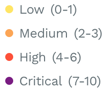Impact Risk Level = Low (0-1), since

0<=0<=1Risk Score = Grid cell corresponding to High Likelihood and Low Impact = 3 as shown in the lookup table below.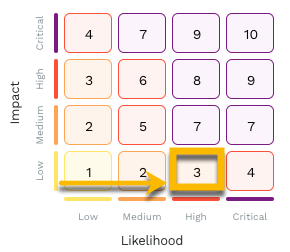Risk Level = Medium (2-3) because the score is 3.Example 2 has the following assumptions for the API:

• The API is external - You can sort APIs based on External or All () filter from the API Endpoints page.
• API is popular, contains 6 request parameters and has 4 PII data in response.
• The API is tagged as sensitive.
LikelihoodImpact

Total number of factors = 3 (Motive is disabled)

Total number of factors = 3

• Exploit Surface Factor score = maximum of matched elements = 7
• Matched Request has 2 or more params Hence score = 3
• Matched Request has 5 or more params: Hence score = 7
• Ease of Access Factor score = maximum of matched elements = 10
• Matched Endpoint is external and popular: Hence score = 10
• Tags Factor score = maximum of matched elements = 0
• Matched Sensitive: Score = 0
• Sensitive Data Exfiltration Factor score = maximum of matched elements = 8
• Matched Response contains PII >= 3: Hence score = 8
• Loss of Confidentiality Factor score = 0 (no elements matched)
• Tags Factor score = maximum of matched elements = 8
• Matched Sensitive: Hence score = 8

Likelihood score = (Sum of factor scores)/(Total no. of factors) = (7+10+0)/3 = 5.67

Impact score = (Sum of factor scores)/(Total no. of factors) = (8+0+8)/3 = 5.33

Likelihood Risk Level = High (4-6), since 4<=5.67<=6Impact Risk Level = (4-6), since 4<=5.33<=6